# Running Snake on WCH Chitu RISC-V MCU

Author Edwin

This tutorial will use the LCD and Five to switch modules on the Chitu development board to implement "Play Snake with Chitu". The code is open sourced at: https://gitee.com/verimaker/opench-chitu-game-demos

In order to provide a better introduction to the development process, we will explain the design process in two parts:

1. The related API used by LCD module; the simple use of the Five to switch.
2. Data structure design and algorithm implementation of Snake.

### 1、Introduction of LCD module and Five to switch

#### 1.1 LCD

There is a small 240*240 TFT screen on the Chitu development board. The control chip of the screen is ST7789, which can use the SPI interface or the 8080 interface, and the Chitu development board uses the 8080 interface.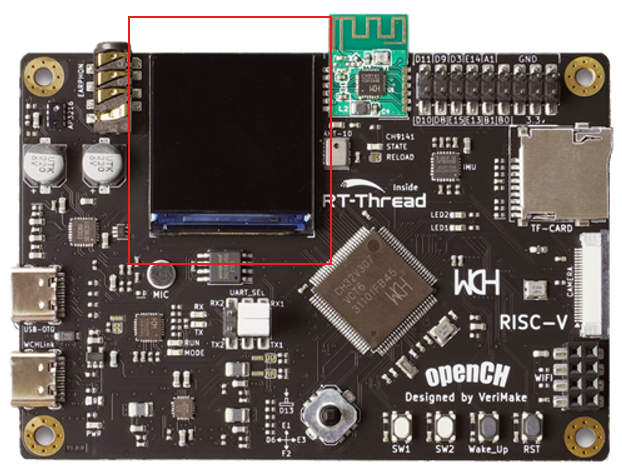In order to be able to use this TFTLCD simply, we encapsulated the configuration and operation of this LCD into a series of APIs, which are stored in lcd.c and lcd.h, and the fonts of English and numbers are placed in font.h , which stores three sizes of fonts are 32, 24 and 12 sizes. You only need to #include "lcd.h" when using it. Now, let's take a look at the related functions and their use.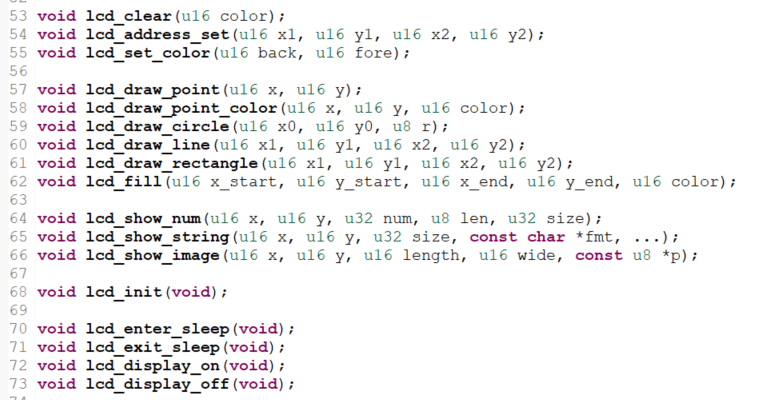The following describes the commonly used functions.
lcd_init(); //The lcd initialization function needs to be executed once at the beginning of the program. It mainly configures the relevant ports and functions of the lcd.

After configuration, it can display strings, numbers, fill color blocks, draw points, lines, rectangles, circles, etc. on the lcd. For example, draw a point lcd_draw_point(u16 x, u16 y); draw a point at coordinates x, y, the color of this point is determined by lcd_set_color(u16 back, u16 fore); You can also use lcd_draw_point_color(u16 x, u16 y, u16 color) in one step, note that color is set in RGB565 format.

Other functions, as their names suggest, are relatively easy to understand, If you don't understand, you can try it out and see what is displayed on the screen. Another point to note is the coordinate relationship on the screen corresponding to the screen coordinates x and y. The upper left corner of the screen in the LCD configuration we provide is the origin of the display coordinates. Horizontally to the right is the positive direction of x, that is, x is the column coordinate. Down is the positive y direction, that is, y is the row coordinate.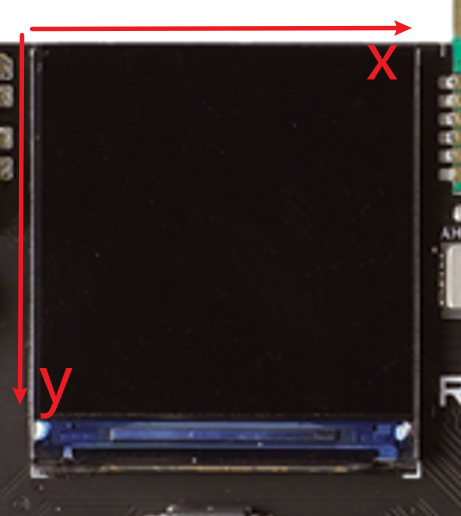For example, if we want to display a red dot of one pixel in the middle of the screen, we only need to copy lcd.c, lcd.h and font.h to the new project directory user, and then add the initial function and the drawing point function to the main function.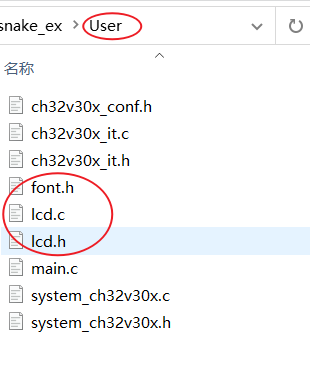The code in the main function is as follows: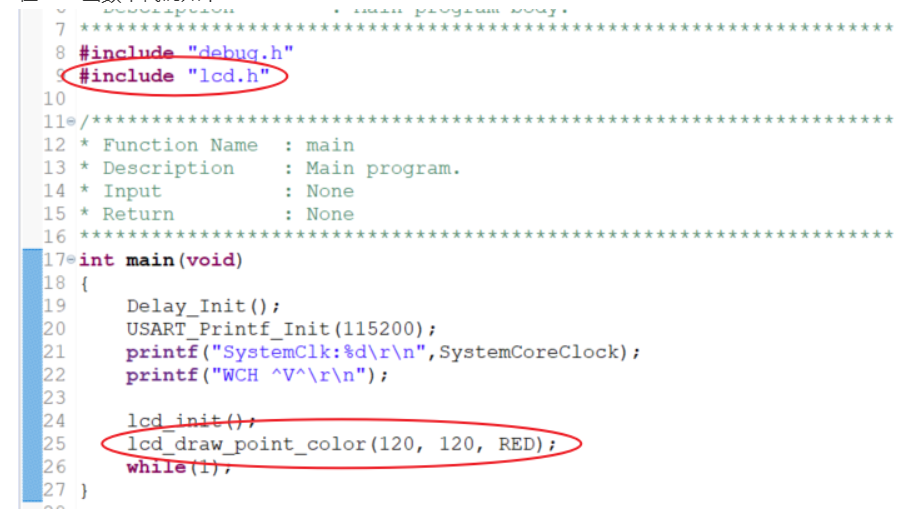For all the other functions it is possible to try to display the effect in this way. A very small red dot in the center can be seen in the video demo, which shows that the pixels displayed on the screen are still very small, so we need to use multiple pixels to display the structure of the snake when we design the it later.

#### 1.2 Five-phase rocker switch

We also need a controller to control the trajectory of the snake when we are playing the game. A five-phase switch is configured on the Chitu development board, which can input up, down, left, right, and press five actions. Looking up the port correspondence table of the Chitu development board, we can see that these five directions are connected to the five IO ports of the 307 respectively.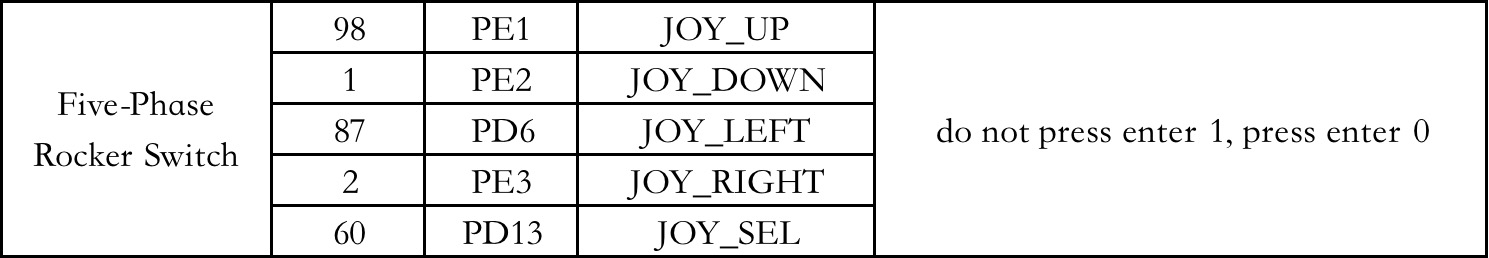The method of detecting the switch can use the button interrupt or query the IO port status. Here we use the query IO port status method to check the switch status, and design a function to return the queried button value.

Before designing the function, we need to set the relevant IO to input and turn on the relevant peripheral clock.

void GPIO_INIT(){
GPIO_InitTypeDef GPIO_InitTypdefStruct;

RCC_APB2PeriphClockCmd(RCC_APB2Periph_GPIOA|RCC_APB2Periph_GPIOD|RCC_APB2Periph_GPIOE,ENABLE);

GPIO_InitTypdefStruct.GPIO_Pin = GPIO_Pin_1|GPIO_Pin_2|GPIO_Pin_3|GPIO_Pin_4|GPIO_Pin_5;
GPIO_InitTypdefStruct.GPIO_Mode = GPIO_Mode_IPU;
GPIO_InitTypdefStruct.GPIO_Speed = GPIO_Speed_50MHz;
GPIO_Init(GPIOE, &GPIO_InitTypdefStruct);

GPIO_InitTypdefStruct.GPIO_Pin = GPIO_Pin_0;
GPIO_InitTypdefStruct.GPIO_Mode = GPIO_Mode_IPU;
GPIO_InitTypdefStruct.GPIO_Speed = GPIO_Speed_50MHz;
GPIO_Init(GPIOA, &GPIO_InitTypdefStruct);

GPIO_InitTypdefStruct.GPIO_Pin = GPIO_Pin_6|GPIO_Pin_13;
GPIO_InitTypdefStruct.GPIO_Mode = GPIO_Mode_IPU;
GPIO_InitTypdefStruct.GPIO_Speed = GPIO_Speed_50MHz;
GPIO_Init(GPIOD, &GPIO_InitTypdefStruct);
}

Here we have initialised both buttons as well.
The following is the query function design of the button.

#define up      1
#define down    2
#define left    3
#define right   4
#define sel     5
#define sw1     6
#define sw2     7

/*******************************************************************************
* Function Name  : Basic_Key_Handle
* Description    : Basic Key Handle
* Input          : None
* Return         : 0 = no key press
*                  key = key press down value
*******************************************************************************/
uint8_t Basic_Key_Handle( void )
{
uint8_t keyval = 0;
if( ! GPIO_ReadInputDataBit( GPIOE, GPIO_Pin_4 ) )
{
Delay_Ms(20);       //  Delayed de-shaking
if( ! GPIO_ReadInputDataBit( GPIOE, GPIO_Pin_4 ) )
{
keyval = sw1;
}
}
else {
if( ! GPIO_ReadInputDataBit( GPIOE, GPIO_Pin_5 ) )
{
Delay_Ms(20);
if( ! GPIO_ReadInputDataBit( GPIOE, GPIO_Pin_5 ) )
{
keyval = sw2;
}
}
else {
if( ! GPIO_ReadInputDataBit( GPIOE, GPIO_Pin_1 ) )
{
Delay_Ms(20);
if( ! GPIO_ReadInputDataBit( GPIOE, GPIO_Pin_1 ) )
{
keyval = up;
}
}
else {
if( ! GPIO_ReadInputDataBit( GPIOE, GPIO_Pin_2 ) )
{
Delay_Ms(20);
if( ! GPIO_ReadInputDataBit( GPIOE, GPIO_Pin_2 ) )
{
keyval = down;
}
}
else {
if( ! GPIO_ReadInputDataBit( GPIOE, GPIO_Pin_3 ) )
{
Delay_Ms(20);
if( ! GPIO_ReadInputDataBit( GPIOE, GPIO_Pin_3 ) )
{
keyval = right;
}
}
else {
if( ! GPIO_ReadInputDataBit( GPIOD, GPIO_Pin_6 ) )
{
Delay_Ms(20);
if( ! GPIO_ReadInputDataBit( GPIOD, GPIO_Pin_6 ) )
{
keyval = left;
}
}
else {
if( ! GPIO_ReadInputDataBit( GPIOD, GPIO_Pin_13 ) )
{
Delay_Ms(20);
if( ! GPIO_ReadInputDataBit( GPIOD, GPIO_Pin_13 ) )
{
keyval = sel;
}
}
}
}
}
}
}
}

return keyval;
}

This function queries the value of the currently pressed key, returns the value corresponding to the key, or returns 0 if no key is pressed.

### 2、Data Structure Design and Algorithm Implementation of gluttonous Snake

#### 2.1 The design of snake

To design Snake. First, according to the rules of the game, we need a controllable snake and one or more random food. The snake can move up, down, left and right under our control. After swallowing food, the snake body will grow one part. This seems to be a relatively complicated thing. If some rules or gameplay are added, it will be more complicated, but the entertainment experience will be better. For example, you can design obstacles on the screen; design different food to have different effects after swallowing, etc.

Let's start with a simple process, sort out the process first, which will help us to design. The flow chart of gluttonous snake is as follows: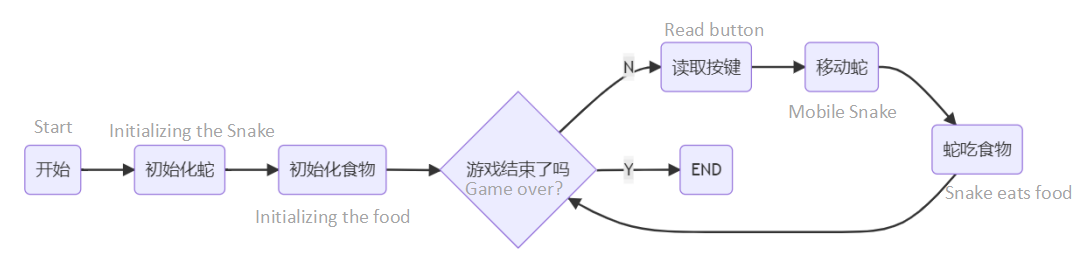So how to describe a snake? The snake has three sections: head, body and tail. To display a snake on the LCD, you only need to know the coordinates of the center point of each section. To simplify, we can use an 8*8 pixel grid to represent part of the snake, which only one square area needed to be filled on the LCD. For example, if you want to display a snake head (represented by a red square) in the center of the screen (the center coordinates are 120, 120), you only need to use lcd_fill(120-4,120-4,120+4,120+4,RED);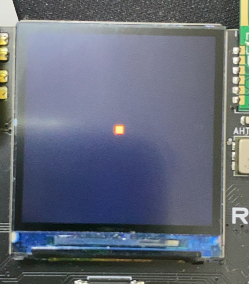The snake body and tail can be distinguished by different colors, and the connecting part can be displayed with two blank lines. Each part of the snake actually only needs to store its center coordinates. So how to store this snake? What kind of data structure is used to store it? Since the length of the snake will get longer and longer as the snake eats the food, if you use an array to store it, the size of the array cannot be determined, because the length of the snake is uncertain, and if you define a large array at the beginning, it will waste memory. The correct way is to use a structure to hold the snake's coordinates, and a linked list to link each part of the snake. The snake structure is defined as follows:

typedef struct Snakes
{
int x;
int y;
struct Snakes *next;
}snake;

snake *head;  // Define snakehead pointer

The pointer in the snake head structure points to the structure of the snake body, and the pointer in the snake body points to the snake tail structure, thus forming a very intuitive snake structure:The pointer in the snake tail points to NULL, which is also the sign of the snake tail.

Let's create a snake, initialize the snake in a fixed position. For example, the center coordinates of the snake head are set to (18, 18), what should be the coordinates of the snake body and tail? Since the snake is an 8*8 square, and the joints occupy two pixels, the distance between the snake head and the snake body should be 10 pixels, and is the same as the snake body and snake tail. So if you want the snake to be vertical when it is initialized, the coordinates of the center of the snake's body are (18,28), and the coordinates of the snake's tail are (18,38). The code to create a snake is as follows:

void creatsnake(){
snake *body,*tail;
head = (snake *)malloc(sizeof(snake)); //Let the head of the snake point to a memory
body=(snake *)malloc(sizeof(snake));// the snake body pointer point to a memory
tail=(snake *)malloc(sizeof(snake));//the snaketail pointer point to a memory

head->x=18;  //Initialize the initial coordinates of the snake
body->x=18; //Snake body coordinates
body->y=28;
tail->x=18; //Snaketail coordinates
tail->y=38;

head->next=body; //the snakehead points to the body of the snake
body->next=tail; //the snake body points to the snaketail
tail->next=NULL; //the snaketail points to NULL
}

In this way, the data of the snake has been initialized in memory. But nothing can be seen on the LCD because the snake is not printed on the screen. The function that prints the snake is as follows:

void printsnake(){
uint8_t flag =0;
while(p->next!=NULL){
if(flag == 0){
lcd_fill((p->x)-4,(p->y)-4,(p->x)+4,(p->y)+4,RED);
flag++;
}
else {
//body
lcd_fill((p->x)-4,(p->y)-4,(p->x)+4,(p->y)+4,BLUE);
}
p=p->next;
}
//tail
lcd_fill((p->x)-4,(p->y)-4,(p->x)+4,(p->y)+4,GREEN);
}

In this function, first print the snake head, then traverse the entire snake body to print the snake body, and finally print the snake tail, so that no matter how long the snake is, it can be printed out.

The display is as follows: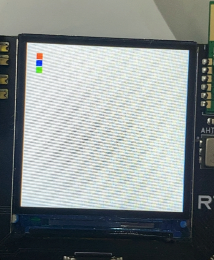The head is red, the body is blue, and the tail is green.

#### 2.2 Control the movement of the snake with a five-way switch

How does the snake move? This is the most critical algorithm in the design of this project. On the data, the center coordinate of the snake head will be added or subtracted 10 in x or y in the direction of movement, the same as the snake body and tail. If so, the snake’s head, body and tail all need to be calculated. When the snake body is short, the amount of calculation is not big. What if the snake body is very long? So here, the smallest calculation amount is the best algorithm. We provide a simple algorithm: first, we know the moving direction of the snake according to the value obtained by the button, create a new snake head in the moving direction of the snake, and connect this snake head to the old snake head as shown in the figure: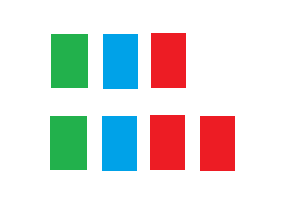Then through the traversal, it will remove the tail, turning the last section of the original snake body into the tail. The process is as follows:Remove the middle part and get the following picture as follows: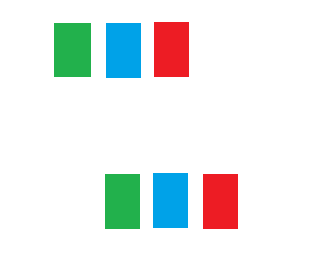As can be seen from the figure, the snake moves forward by one grid, and only the coordinates of the snake head increase one grid in the direction of movement, and the coordinates of the snake body and the snake tail do not need to be calculated. Suppose we let the snake move to the right fixedly, in order to control the speed of the snake's movement, add a delay between each movement. The code is as follows:

void movesnake(){
snake *p,*q;

x+=10;  //fixed rightward movement
snake *newhead=(snake *)malloc(sizeof(snake)); //create a new snakehead node
newhead->next = head;    //attaching the new snake head to the old one
lcd_fill((q->x)-4,(q->y)-4,(q->x)+4,(q->y)+4,BLUE);  //print the original snake head into a snake body
while(p->next!=NULL){
q=p;
p=p->next;
}                        //move to the tail of snake
lcd_fill((p->x)-4,(p->y)-4,(p->x)+4,(p->y)+4,WHITE);//Remove the tail of the snake and insert it.
q->next=NULL;
lcd_fill((q->x)-4,(q->y)-4,(q->x)+4,(q->y)+4,GREEN);//print new snake tail
free(p);
Delay_Ms(250);     //delay
}

Add movesnake() to while(1) in the main function, download it to the Chitu development board, let's see what happens:Next, let the snake move according to the switch instructions . The switch has four directions: up, down, left and right. It should be noted that the snake actually only has three directions to move: forward, left or right, and no backward. Therefore, it is necessary to control the limitation of switch judgment. In addition, remember to add the function retrieved by the previous switch to main.c. The snake moving code is added to the following form:

uint8_t dir = 0;  //use a global variable to store the current direction of the snake's movement
uint8_t key = 0;

void movesnake(){
uint8_t getkey =0;
snake *p,*q;

getkey = Basic_Key_Handle();  //read key values
if (getkey) {
key=getkey;    //updates the key value if a key is pressed, maintains the original movement if no new key is pressed
}

switch (key)
{
case up:
if (dir!=down) {// you cannot turn directly upwards when moving downwards, as with the following
y-=10;
dir = up;
}
break;
case down:
if (dir!=up) {
y+=10;
dir = down;
}
break;
case left:
if (dir!=right) {
x-=10;
dir = left;
}
break;
case right:
if (dir!=left) {
x+=10;
dir = right;
}
break;
default:
break;
}
if(x!=head->x||y!=head->y){ //keep the original motion when no key is pressed
snake *newhead=(snake *)malloc(sizeof(snake)); //create a new snakehead node
lcd_fill((q->x)-4,(q->y)-4,(q->x)+4,(q->y)+4,BLUE);  //print the original snake head into snake body
while(p->next!=NULL){
q=p;
p=p->next;
}                        //move to snake tail.
lcd_fill((p->x)-4,(p->y)-4,(p->x)+4,(p->y)+4,WHITE);//remove the snake tail, and insert the snake tail.
q->next=NULL;
lcd_fill((q->x)-4,(q->y)-4,(q->x)+4,(q->y)+4,GREEN);//print the new snake tail.
free(p);
Delay_Ms(250);     //delay
}
}

In this way, you can use the five-way switch to control the movement of the snake.

#### 2.3 Feed the snake

We finally move to the part of snake eating food. First, we need to have food, the food that appears on the screen is actually a coordinate (the center coordinate of the food). So how to design the data structure of food? In fact, it is very simple and only needs a structure to store the center coordinates of the food.

struct Foods
{
int x;
int y;
}food;

Here we design the food to be an 8*8 pixel square (to facilitate the collision detection design later).The coordinates of the food should be random, so that it is in line with the fun of the game. If you want the x and y of the coordinates to be random numbers, we can use a random number generator peripheral (hardware random number generator) inside the CH32V307. Refer to Chapter 29 Random Number Generator (RNG) chapter of CH32V307 data sheet, a random number can be obtained through simple configuration. First you need to add the header file #include "ch32v30x_rng.h", then by turning on the random number peripheral clock, and then enabling it, you can get the random number. Add the initialization code to the main function as follows:

RCC_AHBPeriphClockCmd(RCC_AHBPeriph_RNG, ENABLE);
RNG_Cmd(ENABLE);

Obtained by the function RNG_GetRandomNumber() where random numbers are required.

We need to design the range of random occurrences of food. In general, these are the requirements：

1. The random coordinates must be within the screen display range, which is 0-239.

2. Food cannot appear on any part of the snake's body.

3. Food must be on the road that the snake can travel through.

The first two requirements are easy to understand, the third requirement is that If you need to design snake head and food collision determination, you can use a variety of methods, one of the simplest methods is center coordinate collision. In this case, since the movement of the snake is a movement of plus or minus 10, that is, a change of 10 at a time, if the food center happens to appear in the middle of the two roads, then the snake will never be able to eat this food. If we use edge collision determination, we can solve this problem, but the algorithm will be complicated. We will explain the easy-to-understand and simple center collision as an example. We need the random number generated randomly within 0-239, and then in order not to let the snake go beyond the screen. We first need to design a screen range. Here, we design rows 0-3 and columns 0-3 as screen borders, and also leave 4 rows and 4 columns at the left and bottom as screen borders. In this way, the snake walking path is 8, 18, 28..., the maximum is 228. Just need a random number between 0-22, then multiply by 10 and add 8, the ranks are the same.

((RNG_GetRandomNumber())%(23))*10+8;

Next, create the food function when writing initialization, and implement the algorithm requirements of requirement 1 and 2 in it.

void creatfood(){
int flag=0; //create a food success sign, 1 for success
while(!flag){  //until the food is created
flag=1;
food.x=((RNG_GetRandomNumber()%23)*10+8);//generate random coordinates, and on the snake's walking path
food.y=((RNG_GetRandomNumber()%23)*10+8);

snake* judge = head;
while (1)  //traverse the body of the snake to find out if the food produced is on the body of the snake
{
if (food.x == judge->x && food.y == judge->y)
{
flag = 0;  //if on the snake it means the creation failed
}
if (judge->next == NULL) break;  //if the end of the snake is reached, exit the loop
judge = judge->next;
}
}
lcd_fill(food.x-4, food.y-4, food.x+4, food.y+4, RED); //if the creation is successful, print the food to the screen
}

Add the creation of food to the main function to initialize creatfood(); download it to the Chitu development board, and you will see the food appear in different positions every time you restart.

#### Eat food

In the previous description, we design the food, and this food center is on the center moving path of the snake. When the coordinates of the snake head and the food are the same, it means that the snake has eaten the food. After the snake eating the food, the food will disappear and a new food will be regenerated. In addition, the snake's body is increased by 1 grid. According to this requirement, an algorithm is designed as follows:

void eatfood(){
if (head->x == food.x && head->y == food.y) //if the snake head and food coordinates collide
{
creatfood();//create a new food
snake* _new = (snake*)malloc(sizeof(snake)); //create a new snake body
snake* p;
while (1)   //traverse to snake tail
{
if (p->next == NULL) break;
p = p->next;
}
p->next = _new;  //add the new body to the tail and connect it
_new->next = NULL;
}
}

Then put this function in the while inside the main function.

while(1){
movesnake();
eatfood();
}

Download the code to the Chitu development board, then you can implement the snake eating food.

#### 2.4 Game judgment

So far, the process of Snake has been completed, but no matter how it goes or eats, the game will not be GAME OVER! Because we have not yet judged the success or failure of the game, the while judgment in the main function is 1, and it will continue to loop. Now we want to add the game's success or failure decision, replace the decision 1 in the while, and make it a complete game.

First we devise a few rules for determining the game: 1. The snake cannot go beyond the map; 2. The snake cannot bite its own body or tail. As soon as one of these happens the game is over.

Let's add a decision function to implement the above two rules

int judge(){
return 0;
}
while(p->next!=NULL){
if(head->x==p->x&&head->y==p->y){//check for bites on the snake's body
return 0;
}
p=p->next;
}
if(head->x==p->x&&head->y==p->y){//check for bites on the snake's tail
return 0;
}
return 1;
}

Replace the while in main with:

while(judge()){
movesnake();
eatfood();
}

If the game is over, show the classic GAME OVER!

Add after the end of while:

while(judge()){
movesnake();
eatfood();
}
lcd_show_string(50, 120, 32, "GAME OVER");

Now the simple version of Snake has completed.

### One More Thing

If you want to make the game more interesting, you can add some functions in the corresponding modules, like: in the eat food function, you can increase the statistics of scores, and you can also increase the difficulty of the game according to the increase of scores, for example, every time there is more food, more obstacles, etc. You can also use the network port expansion board of the Chitu to realize the network connection of the Chitu development board to realize the Snake of the network battle.